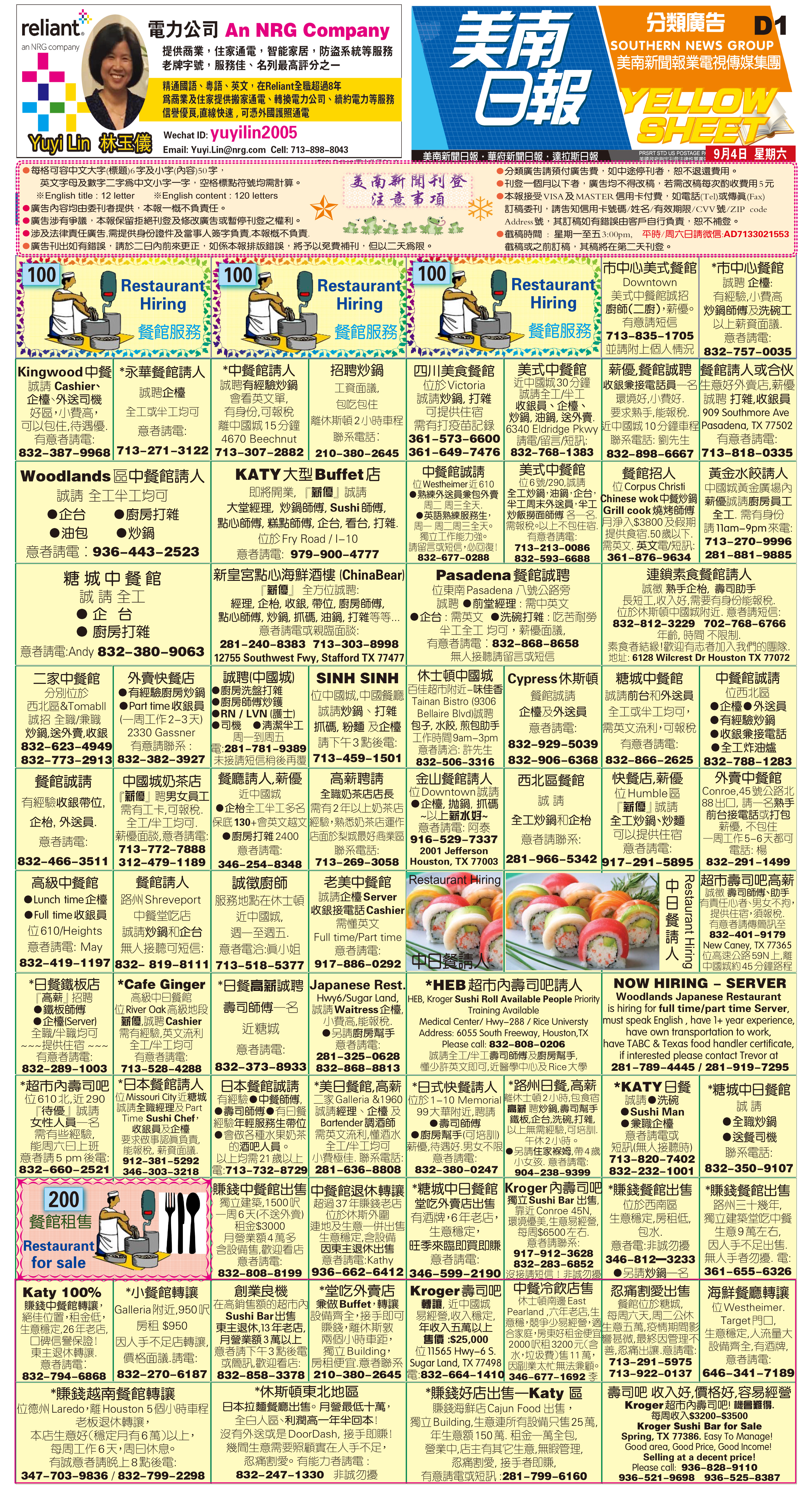210917 Epaper

 A Section B SectionC Section D Section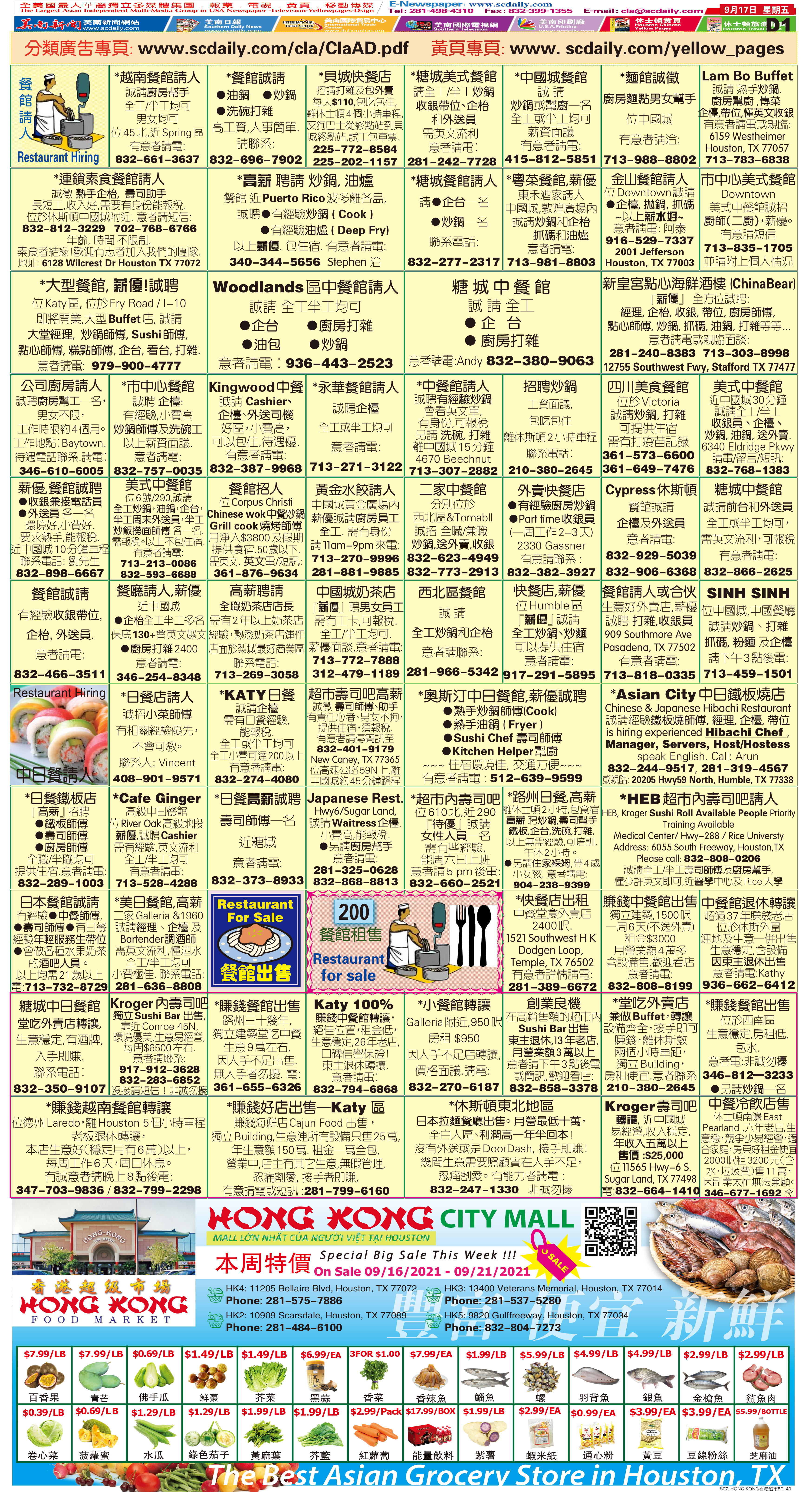210916 Epaper

 A Section B SectionC Section D Section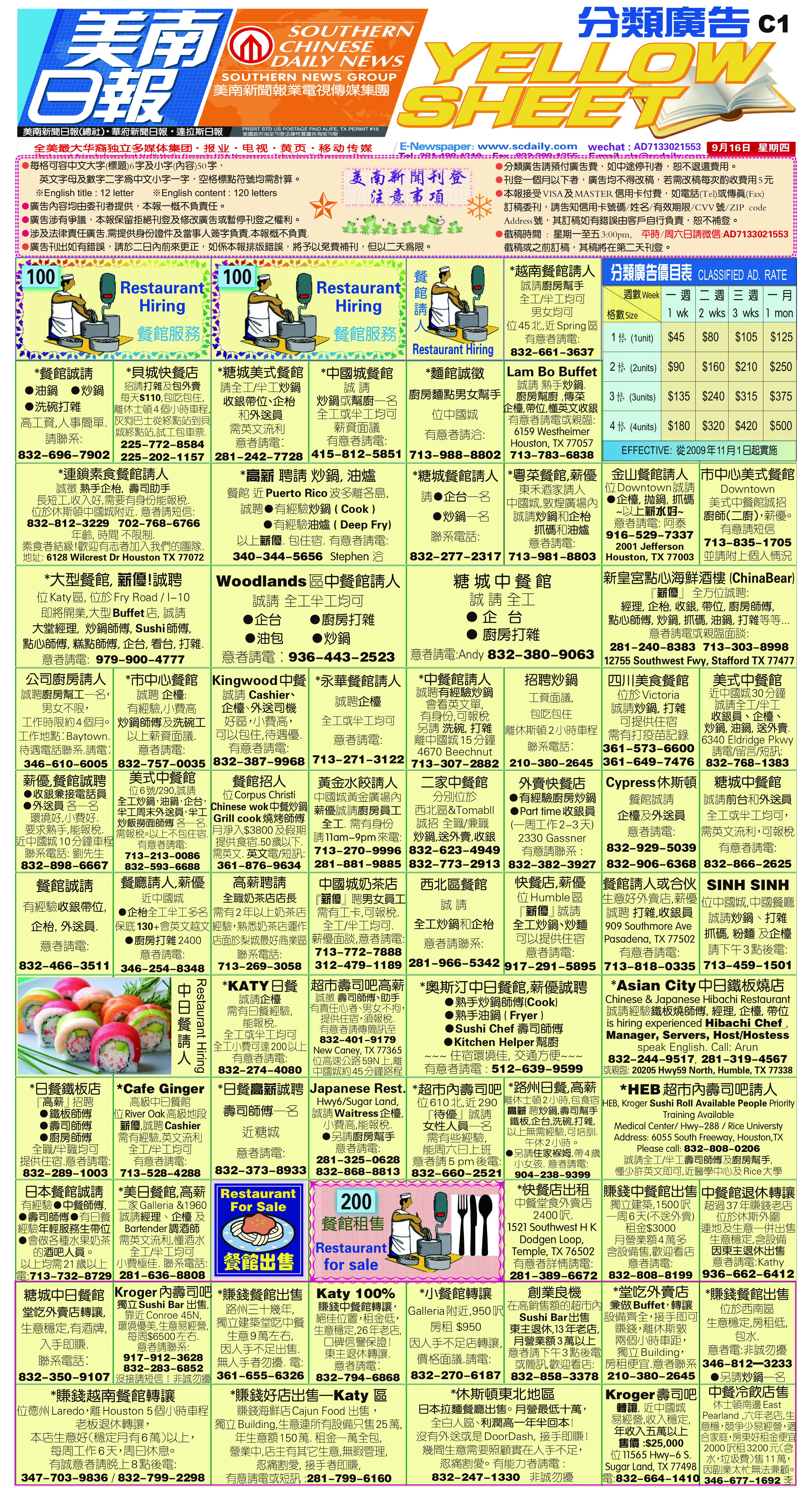210915 Epaper

 A Section B SectionC Section D Section210914 Epaper

 A Section B SectionC Section D Section210913 Epaper

 A Section B SectionC Section D Section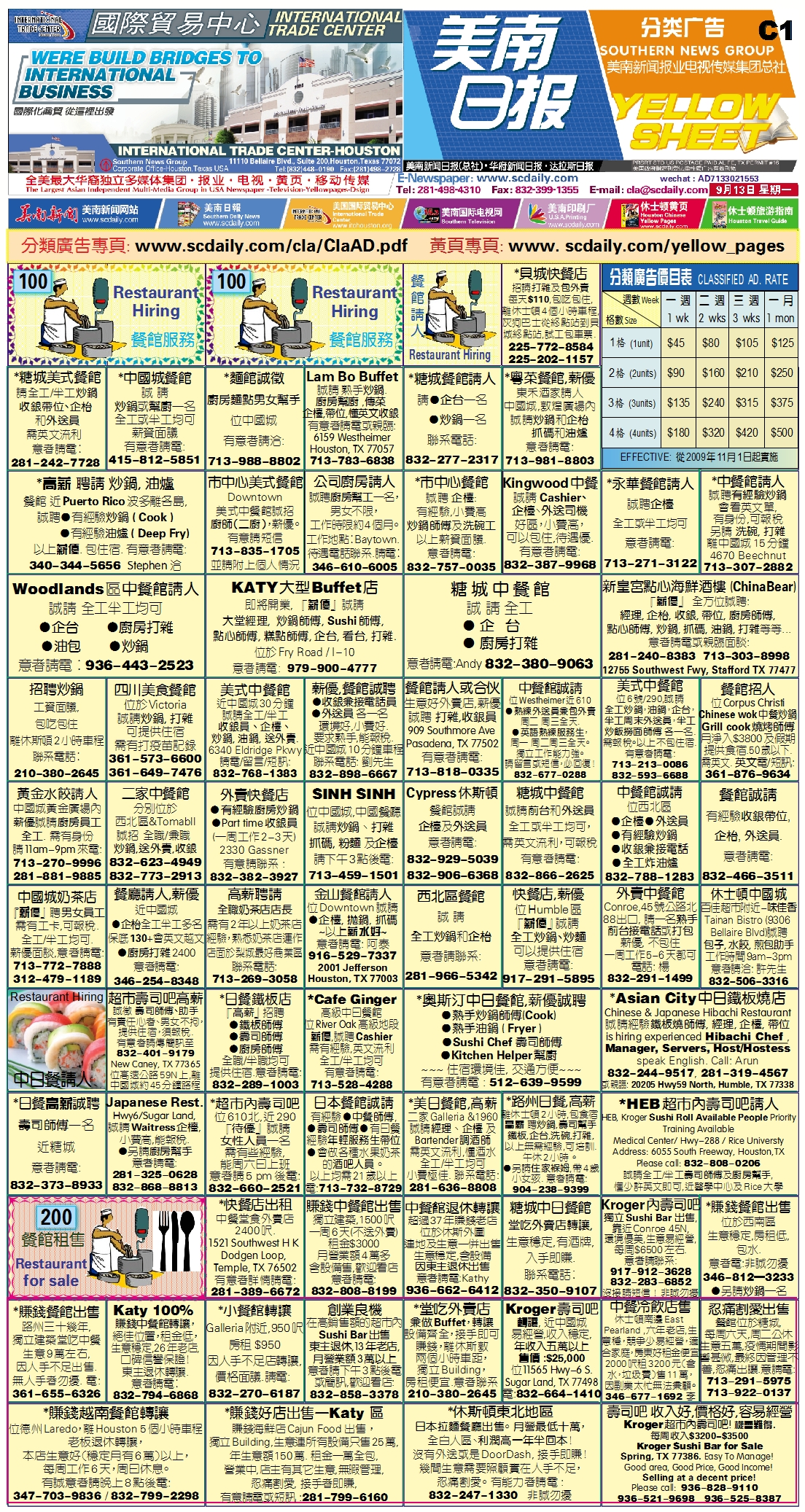210912 Epaper

 A Section B SectionC Section D Section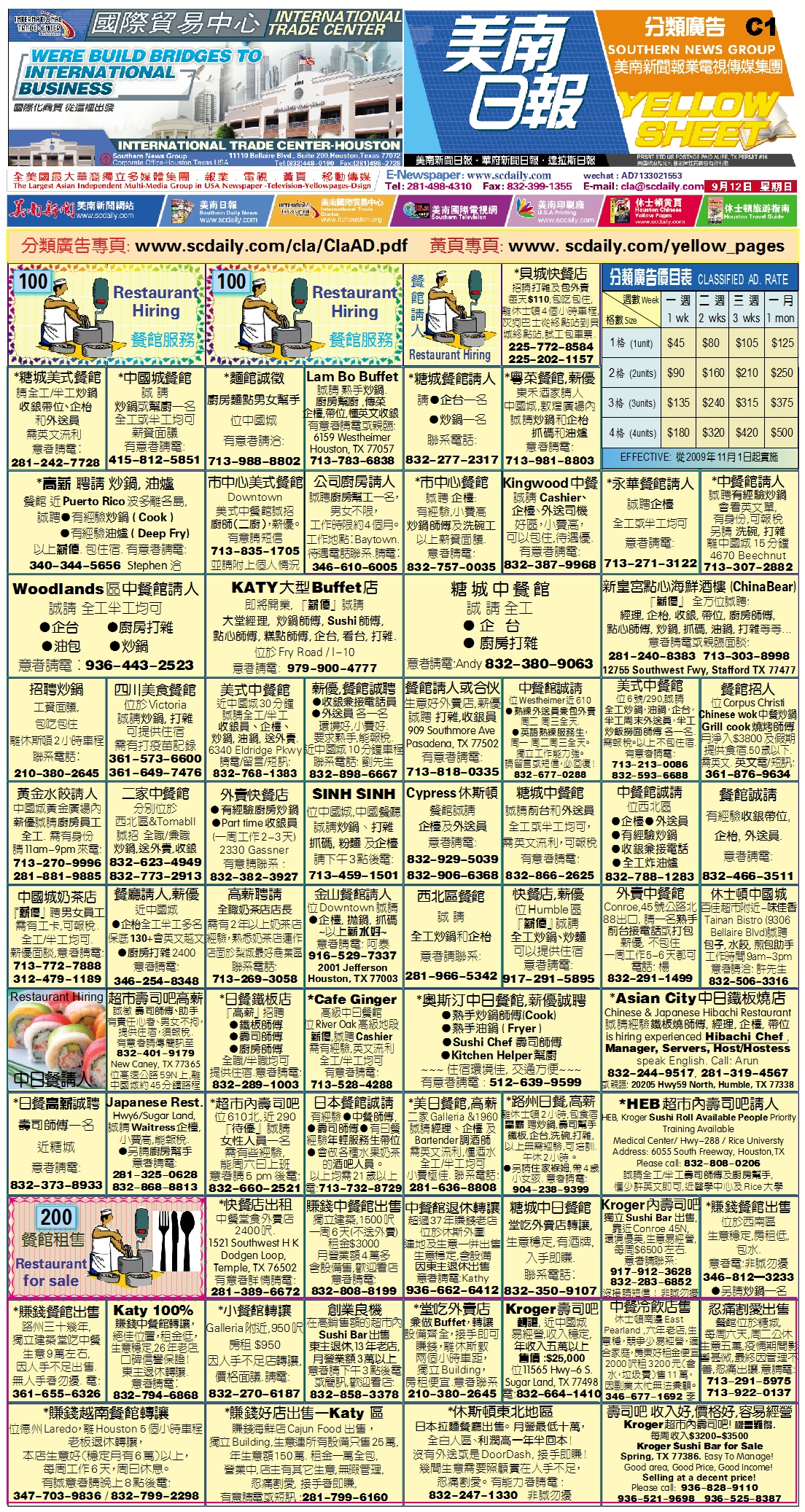210911 Epaper

 A Section B SectionC Section D Section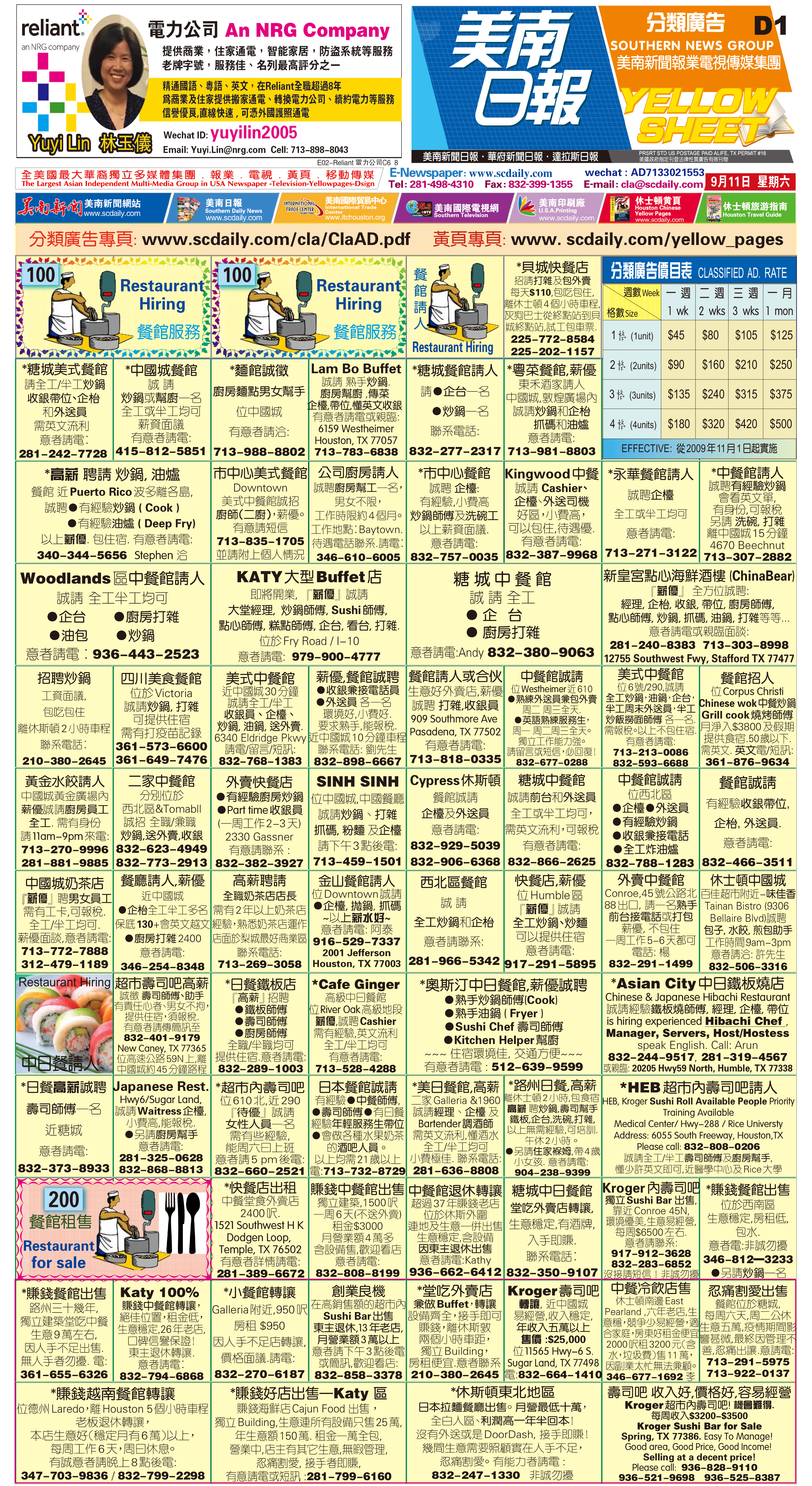210910 Epaper

 A Section B Section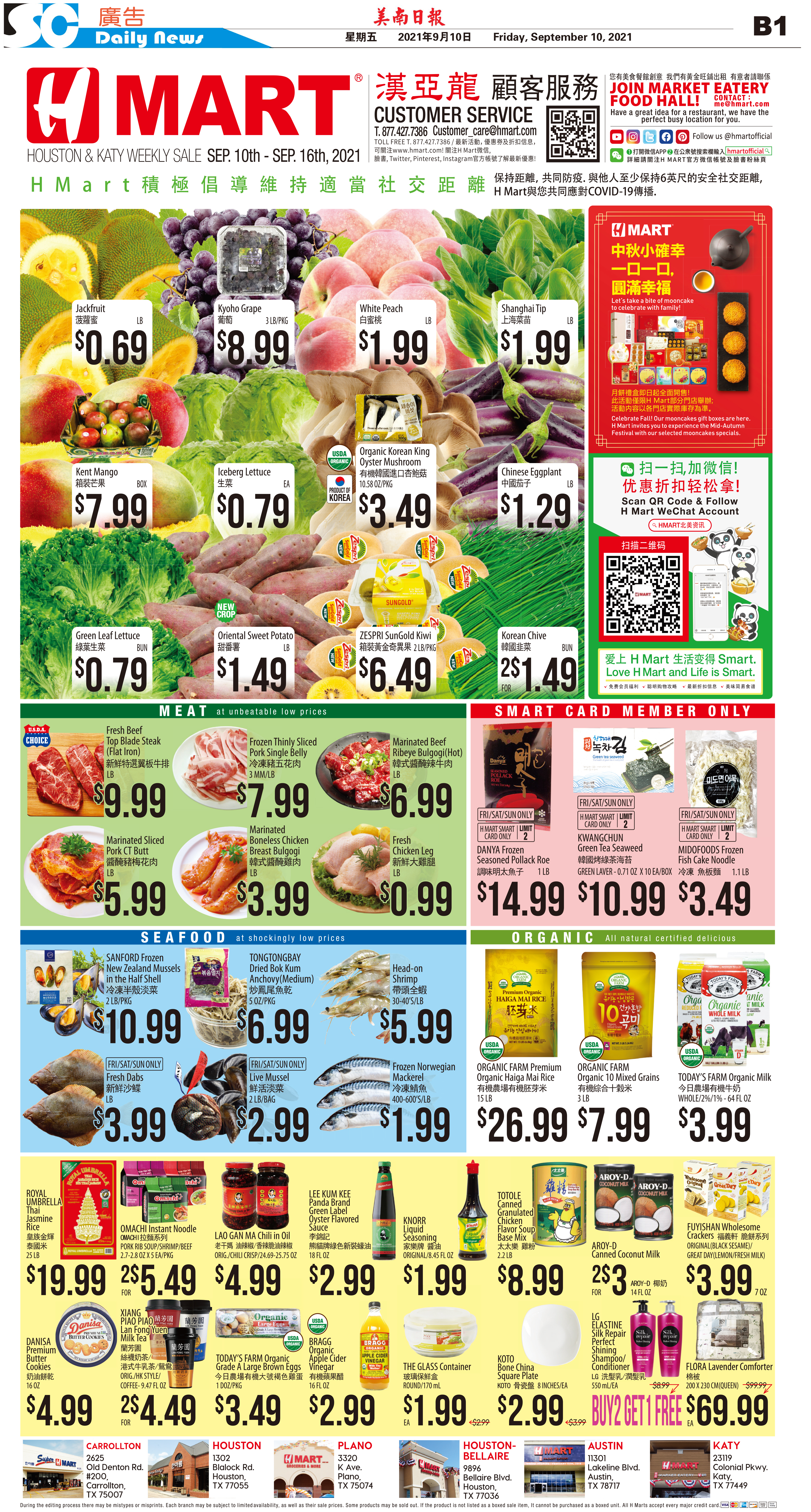C Section D Section210908 Epaper

 A Section B SectionC Section D Section210909 Epaper

 A Section B SectionC Section D Section210904 Epaper

 A Section B SectionC Section D Section